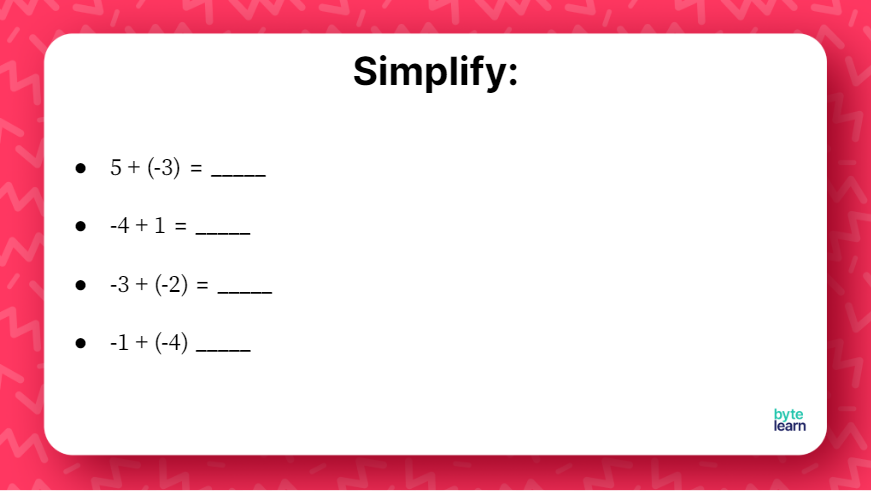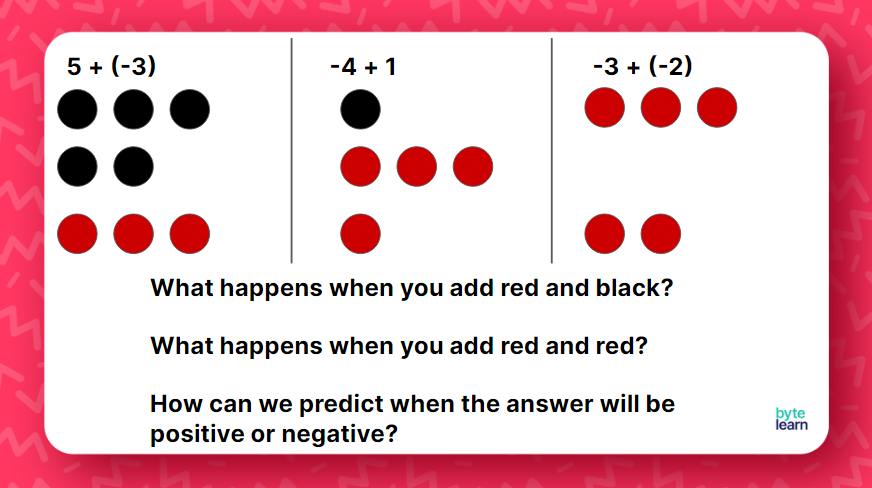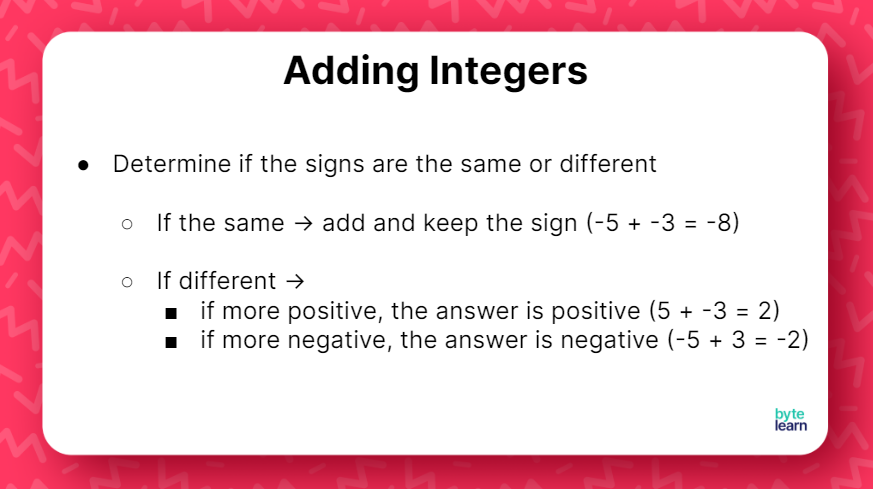ResourcesTestimonials
Plans
ResourcesTestimonials
PlansLesson plan

## Overview

In this lesson, students will learn how to add integers without manipulatives. After reviewing visual models, students will practice applying the rules for values with the same sign or different signs. You can expect this lesson with additional practice to take one 45-minute class period.

Signed Number Operations
7.NS.A.1.D

## Objective

Students will be able to add integers.

## Materials

• Teacher Slideshow
• Counters or algebra tiles
• Online Practice

## How to Teach Adding Integers

### Warm-up

Before this lesson, it is recommended that you spend a day with visual models for students. You can use this lesson for the first day of introducing adding integers!

For this lesson, it will be helpful if you still have counters or algebra tiles that have different colored sides to help students better understand how to add integers. If you do not have these available, then students can also draw “+” or “–” symbols or use black and red pencils or pens to differentiate between positive and negative values.### Discuss with the class

When reviewing with students, make sure students can explain how they determined the values of each model. Students should recognize that one red and one black cancel each other out. Reinforce the vocabulary for “zero pairs” if needed.

### Reviewing with integer counters

Although the goal is that students will not have to rely on counters or algebra tiles to add integers, they can be beneficial in helping students concretely understand the rules for adding integers.Make sure students have counters, algebra tiles, or colored writing utensils if needed to help them simplify each expression. When reviewing the answers, you can use the following slide to give visual representations for the first three questions.Although the fourth question is not shown visually, the purpose of these questions is to help students discover the rules for adding integers. Allow students time to discuss what happens when they add red and black or red and red to help them predict when their answers will be positive or negative. Then, when reviewing the fourth question, students can use their predictions to see if they were right.

To help students solidify their understanding of adding integers, review that students must determine if the signs of the values are the same or different.If the values have the same sign, they can just add and keep the original sign both numbers had. If the values have different signs, students can subtract the values and keep the sign of the number with a higher absolute value (or farthest from zero).

For this example, ideally, students will be able to recognize that the values have the same sign. Because of this, students can just add the values and keep the negative sign. If students answer 5 or -5, then they subtracted the absolute values when they did not need to.

If students are stuck, ask them to picture what these values would look like represented with counters. Students should recognize that they would all be the same color. If needed, students can use the counters or draw “+” and “–” to help represent the values visually; however, students should understand that they will want to work towards not needing those representations.

### Adding a positive to a negative integer

For this next example, students should be able to identify that the signs are different in this example. Ideally, students will know this means they will need to subtract the absolute values and keep the sign of the number with the larger absolute value.

Be mindful of students’ conversations, and make sure you address common misconceptions in calculations, such as adding the values when they should’ve been subtracted.

### Adding a negative to a positive integer

With this last example, students should be able to arrive at the answer without too much assistance. When reviewing the question with students, make sure students can justify how they know the answer should be a negative number.

Before giving students time to work independently, it may be beneficial to have students review the rules for adding integers. Consider having students come up with examples for both cases, where the signs of the values are the same and where the signs of the values are different.